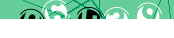# JavaScript: Playable Maze Game Generator

This article combines our previous presented JavaScript Random Maze Generator and Amazing Maze Game into a single package letting you generate playable mazes of different shapes and sizes on demand.

## Working Demonstration

Using the form below you can generate and play randomly generated mazes to your heart's content. There's even an option for enabling speech!

As you navigate the maze, your score goes down with each step, and if it reaches zero, you're out of luck.

On the way there is a key to collect that you will need to open the exit door, and various monsters and treasures that add or subtract points from your score.

Playable Maze Generator

## Source Code

The addition from earlier presented code is the FancyMazeBuilder class which extends the MazeBuilder class with new functions for finessing the maze layout and contents. Otherwise, everything remains as before.

To install the maze on your own page you just need the following code and linked resources:

```<link rel="stylesheet" type="text/css" href="/mazing.css"> <div id="maze_container"><!-- --></div> <script src="/maze-builder.js"></script> <script src="/fancy-maze-builder.js"></script> <script src="/mazing.js"></script> <script> var Maze, MazeGame; const makeMaze = (id, width, height, speech = false) => { Maze = new FancyMazeBuilder(width, height); Maze.display(id); MazeGame = new Mazing("maze"); if(speech) { MazeGame.enableSpeech(); } } makeMaze("maze_container", 12, 12); </script>```

## FancyMazeBuilder Source Code

As before, we're using ES6 class notation which may not be compatible with older browsers.

``` // Original JavaScript code by Chirp Internet: chirpinternet.eu // Please acknowledge use of this code by including this header. class FancyMazeBuilder extends MazeBuilder {   constructor(width, height) {     super(width, height);     this.removeNubbins();     this.joinNubbins();     this.placeSentinels(100);     this.placeKey();   }   isA(value, ...cells) {     return cells.every((array) => {       let row, col;       [row, col] = array;       if((this.maze[row][col].length == 0) || !this.maze[row][col].includes(value)) {         return false;       }       return true;     });   }   removeNubbins() {     this.maze.slice(2, -2).forEach((row, idx) => {       let r = idx + 2;       row.slice(2, -2).forEach((cell, idx) => {         let c = idx + 2;         if(!this.isA("wall", [r, c])) {           return;         }         if(this.isA("wall", [r-1, c-1], [r-1, c], [r-1, c+1], [r+1, c]) && this.isGap([r+1, c-1], [r+1, c+1], [r+2, c])) {           this.maze[r][c] = [];           this.maze[r+1][c] = ["nubbin"];         }         if(this.isA("wall", [r-1, c+1], [r, c-1], [r, c+1], [r+1, c+1]) && this.isGap([r-1, c-1], [r, c-2], [r+1, c-1])) {           this.maze[r][c] = [];           this.maze[r][c-1] = ["nubbin"];         }         if(this.isA("wall", [r-1, c-1], [r, c-1], [r+1, c-1], [r, c+1]) && this.isGap([r-1, c+1], [r, c+2], [r+1, c+1])) {           this.maze[r][c] = [];           this.maze[r][c+1] = ["nubbin"];         }         if(this.isA("wall", [r-1, c], [r+1, c-1], [r+1, c], [r+1, c+1]) && this.isGap([r-1, c-1], [r-2, c], [r-1, c+1])) {           this.maze[r][c] = [];           this.maze[r-1][c] = ["nubbin"];         }       });     });   }   joinNubbins() {     this.maze.slice(2, -2).forEach((row, idx) => {       let r = idx + 2;       row.slice(2, -2).forEach((cell, idx) => {         let c = idx + 2;         if(!this.isA("nubbin", [r, c])) {           return;         }         if(this.isA("nubbin", [r-2, c])) {           this.maze[r-2][c].push("wall");           this.maze[r-1][c] = ["nubbin", "wall"];           this.maze[r][c].push("wall");         }         if(this.isA("nubbin", [r, c-2])) {           this.maze[r][c-2].push("wall");           this.maze[r][c-1] = ["nubbin", "wall"];           this.maze[r][c].push("wall");         }       });     });   }   placeSentinels(percent = 100) {     percent = parseInt(percent, 10);     if((percent < 1) || (percent > 100)) {       percent = 100;     }     this.maze.slice(1, -1).forEach((row, idx) => {       let r = idx + 1;       row.slice(1, -1).forEach((cell, idx) => {         let c = idx + 1;         if(!this.isA("wall", [r,c])) {           return;         }         if(this.rand(1, 100) > percent) {           return;         }         if(this.isA("wall", [r-1,c-1],[r-1,c],[r-1,c+1],[r+1,c-1],[r+1,c],[r+1,c+1])) {           this.maze[r][c].push("sentinel");         }         if(this.isA("wall", [r-1,c-1],[r,c-1],[r+1,c-1],[r-1,c+1],[r,c+1],[r+1,c+1])) {           this.maze[r][c].push("sentinel");         }       });     });   }   placeKey() {     let fr, fc;     [fr, fc] = this.getKeyLocation();     if(this.isA("nubbin", [fr-1,fc-1]) && !this.isA("wall", [fr-1,fc-1])) {       this.maze[fr-1][fc-1] = ["key"];     } else if(this.isA("nubbin", [fr-1,fc+1]) && !this.isA("wall", [fr-1,fc+1])) {       this.maze[fr-1][fc+1] = ["key"];     } else if(this.isA("nubbin", [fr+1,fc-1]) && !this.isA("wall", [fr+1,fc-1])) {       this.maze[fr+1][fc-1] = ["key"];     } else if(this.isA("nubbin", [fr+1,fc+1]) && !this.isA("wall", [fr+1,fc+1])) {       this.maze[fr+1][fc+1] = ["key"];     } else {       this.maze[fr][fc] = ["key"];     }   } } ```

expand code box

Please feed free to adapt and extend these classes as you see fit, and we'd be interested to see what you come up with.

## References

< JavaScript

#### Send a message to The Art of Web:<- copy the digits from the image into this box

press <Esc> or click outside this box to close

```setInterval(function () { var randomColor = Math.floor(Math.random()*16777215).toString(16); document.getElementById("maze").style.backgroundColor = "#" + randomColor; }, 1000);```